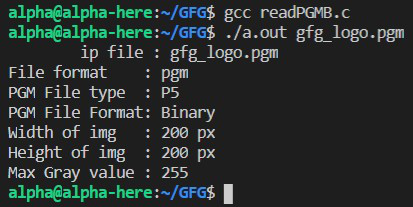# How to read a PGMB format image in C

• Difficulty Level : Hard
• Last Updated : 29 Oct, 2021

PGM or Portable Gray Map file is a grayscale image where each pixel is encoded with 1 or 2 bytes. It contains header information and pixel grayscale values in a matrix form.

Approach: The idea is followed to read PGMB format image is as follows:

Take a step-up from those "Hello World" programs. Learn to implement data structures like Heap, Stacks, Linked List and many more! Check out our Data Structures in C course to start learning today.
• Open a PGMB (binary format PGM image).
• Extract the pixel information, which can be then used for further processing.
• The header information is stored in ASCII format can be read using any text editor but the pixel information is stored in a binary formation and the text editor will show that as some gibberish text.

Below is the sample pgm image.• magic number for identifying the file type:
• Binary PGM File (PGMB): “P5”
• ASCII PGM File (PGMA): “P2”
• Width in ASCII decimal format
• Height in ASCII decimal format
• Maximum Gray Value, ASCII decimal format, between 0-255
• Can contain comments, denoted by beginning the line with a ‘#’
• All separated with white spaces (blanks space, tabs, CRs, LFs)

```P5
# sample PGMB image
# gfg_logo.pgm
200 200
255```

After the header information, there is a grid of dimensions height * weight containing the grayscale pixel values of the image in binary format.

• Open the image in the read binary, rb mode.
• Check if any comments are present and ignore them.
• Read width and height separated by white space.
• Read the max gray value, before and after any white space/comment.
• Read the grid (width * height) of pixel values, separated by white spaces.

Below is the program for the above approach:

## C

 `// C Program to read a PGMB image``// and print its parameters``#include ``#include ``#include ``#include ``#include ` `// Structure for storing the``// image data``typedef` `struct` `PGMImage {``    ``char` `pgmType;``    ``unsigned ``char``** data;``    ``unsigned ``int` `width;``    ``unsigned ``int` `height;``    ``unsigned ``int` `maxValue;``} PGMImage;` `// Function to ignore any comments``// in file``void` `ignoreComments(``FILE``* fp)``{``    ``int` `ch;``    ``char` `line;` `    ``// Ignore any blank lines``    ``while` `((ch = ``fgetc``(fp)) != EOF``           ``&& ``isspace``(ch))``        ``;` `    ``// Recursively ignore comments``    ``// in a PGM image commented lines``    ``// start with a '#'``    ``if` `(ch == ``'#'``) {``        ``fgets``(line, ``sizeof``(line), fp);``        ``ignoreComments(fp);``    ``}``    ``else``        ``fseek``(fp, -1, SEEK_CUR);``}` `// Function to open the input a PGM``// file and process it``bool` `openPGM(PGMImage* pgm,``             ``const` `char``* filename)``{``    ``// Open the image file in the``    ``// 'read binary' mode``    ``FILE``* pgmfile``        ``= ``fopen``(filename, ``"rb"``);` `    ``// If file does not exist,``    ``// then return``    ``if` `(pgmfile == NULL) {``        ``printf``(``"File does not exist\n"``);``        ``return` `false``;``    ``}` `    ``ignoreComments(pgmfile);``    ``fscanf``(pgmfile, ``"%s"``,``           ``pgm->pgmType);` `    ``// Check for correct PGM Binary``    ``// file type``    ``if` `(``strcmp``(pgm->pgmType, ``"P5"``)) {``        ``fprintf``(stderr,``                ``"Wrong file type!\n"``);``        ``exit``(EXIT_FAILURE);``    ``}` `    ``ignoreComments(pgmfile);` `    ``// Read the image dimensions``    ``fscanf``(pgmfile, ``"%d %d"``,``           ``&(pgm->width),``           ``&(pgm->height));` `    ``ignoreComments(pgmfile);` `    ``// Read maximum gray value``    ``fscanf``(pgmfile, ``"%d"``, &(pgm->maxValue));``    ``ignoreComments(pgmfile);` `    ``// Allocating memory to store``    ``// img info in defined struct``    ``pgm->data``        ``= ``malloc``(pgm->height``                 ``* ``sizeof``(unsigned ``char``*));` `    ``// Storing the pixel info in``    ``// the struct``    ``if` `(pgm->pgmType == ``'5'``) {` `        ``fgetc``(pgmfile);` `        ``for` `(``int` `i = 0;``             ``i < pgm->height; i++) {``            ``pgm->data[i]``                ``= ``malloc``(pgm->width``                         ``* ``sizeof``(unsigned ``char``));` `            ``// If memory allocation``            ``// is failed``            ``if` `(pgm->data[i] == NULL) {``                ``fprintf``(stderr,``                        ``"malloc failed\n"``);``                ``exit``(1);``            ``}` `            ``// Read the gray values and``            ``// write on allocated memory``            ``fread``(pgm->data[i],``                  ``sizeof``(unsigned ``char``),``                  ``pgm->width, pgmfile);``        ``}``    ``}` `    ``// Close the file``    ``fclose``(pgmfile);` `    ``return` `true``;``}` `// Function to print the file details``void` `printImageDetails(PGMImage* pgm,``                       ``const` `char``* filename)``{``    ``FILE``* pgmfile = ``fopen``(filename, ``"rb"``);` `    ``// Retrieving the file extension``    ``char``* ext = ``strrchr``(filename, ``'.'``);` `    ``if` `(!ext)``        ``printf``(``"No extension found"``               ``"in file %s"``,``               ``filename);``    ``else``        ``printf``(``"File format"``               ``"    : %s\n"``,``               ``ext + 1);` `    ``printf``(``"PGM File type  : %s\n"``,``           ``pgm->pgmType);` `    ``// Print type of PGM file, in ascii``    ``// and binary format``    ``if` `(!``strcmp``(pgm->pgmType, ``"P2"``))``        ``printf``(``"PGM File Format:"``               ``"ASCII\n"``);``    ``else` `if` `(!``strcmp``(pgm->pgmType,``                     ``"P5"``))``        ``printf``(``"PGM File Format:"``               ``" Binary\n"``);` `    ``printf``(``"Width of img   : %d px\n"``,``           ``pgm->width);``    ``printf``(``"Height of img  : %d px\n"``,``           ``pgm->height);``    ``printf``(``"Max Gray value : %d\n"``,``           ``pgm->maxValue);` `    ``// close file``    ``fclose``(pgmfile);``}` `// Driver Code``int` `main(``int` `argc, ``char` `const``* argv[])``{``    ``PGMImage* pgm = ``malloc``(``sizeof``(PGMImage));``    ``const` `char``* ipfile;` `    ``if` `(argc == 2)``        ``ipfile = argv;``    ``else``        ``ipfile = ``"gfg_logo.pgm"``;` `    ``printf``(``"\tip file : %s\n"``, ipfile);` `    ``// Process the image and print``    ``// its details``    ``if` `(openPGM(pgm, ipfile))``        ``printImageDetails(pgm, ipfile);` `    ``return` `0;``}`

Output:Explanation:

• Create a structure for storing the PGMB image details and allocate memory for the same.
• Take the file name input either as a command-line argument or by hard coding it in the program.
• The openPGM() function processes the input image files and takes the memory pointer and filename are input.
• The ignoreComments() function is used to skip any comments in the file. Comments are usually present in the header portion and thus, we check for them after reading every property.
• In openPGM() function, read the file header information, ie. file type, height, weight, etc as given above.
• Then allocate memory as per the height of the image and for each row, allocate memory for the width of the image.
• The fread() method reads the gray values and stores them in the allocated memory for the 2d character matrix of the pgm structure.
• printImageDetails() is used to print the values retrieved from the PGMB image file.

My Personal Notes arrow_drop_up